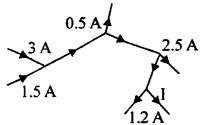# 2nd PUC Physics Series-11

#### PUC Science 2nd Year Physics Series-11 quiz

0
Created on Bywefru

2nd PUC Physics Series 11

1 / 10

101 In following figure shows currents in a part of electrical circuit, then the value of/(in ampere) is given by :2 / 10

102. The smallest resistance that can be obtained by the combination of n resistors each resistance r is :

3 / 10

103. Which of the following has a negative temperature coefficient of resistance ?

4 / 10

104. An electric heating element consumes 500 W, when connected to a 100 V line. If the line voltage becomes 150 V, the power consumed Will be:

5 / 10

Q.105 A uniform w ire connected across a supply produces heat H per second. If wire is cut into three equal parts and all the parts are connected in parallel across the same supply, the heat produced per second will be :

6 / 10

106. n India electricity is supplied fordomestic use at 220 V. It is supplied at 110 V in U.S.A. If the resistance of a 60 W bulb use in India is R. the resistance of a 60 W bulb for use in USA will be:

7 / 10

107. In the following figure represents a balanced Wheatstone bridge circuit. What is the value of X ?8 / 10

108. Two bulbs 25 W, 220 V and 100 W, 220 V are given. Which has higher resistance?

9 / 10

109. Potentiometer measures the potential difference more accurately than a voltmeter because:

10 / 10

110. In a Wheatstone bridge, the resistance each arm is 10 Ω. If the resistance galvanometers is also 10 Ω, then effective resistance of the bridge will be :